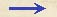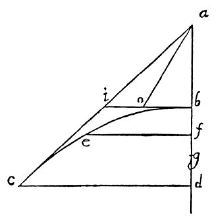Discorsi Propositions 3/04-pr-01 Discorsi Proposition3/04-pr-01PROBLEMA I, PROPOSITIO IV. PROBLEM I, PROPOSITION IVQuomodo datae parabolae, a proiecto descriptae, punctis singulis impetus sit determinandus. To determine the momentum of a projectile at each particular point in its given parabolic path. Sit semiparabola bec, cuius amplitudo cd, altitudo db, quae extensa in sublimi occurrat tangenti parabolam ca in a; et per verticem b sit {30} horizonti et cd parallela bi.Quod si amplitudo cd sit aequalis toti altitudini da, erit bi aequalis ba et bd; et si temporis casus per ab, et momenti velocitatis acquisiti in b per descensum ab ex quiete in a, ponamus mensuram esse ipsammet ab, erit dc (dupla nempe bi) spatium quod per impetum ab, per horizontalem conversum, conficiet eodem tempore: {285} sed eodem tempore cadens per bd ex quiete in b conficit altitudinem bd: ergo mobile cadens ex quiete in a, per ab conversum cum impetu ab, per horizontalem conficit spatium aequale dc.Superveniente vero casu per bd, conficit altitudinem bd, et parabola bc designatur, cuius impetus in termino c est compositus ex aequabili transversali, cuius momentum est ut ab, et ex altero momento acquisito in descensu bd in termino d seu c; quae momenta aequalia sunt.Si ergo intelligamus, ab alterius {10} illorum esse mensuram, ut puta transversalis aequabilis; bi vero, quae ipsi bd est aequalis, esse mensuram impetus acquisiti in d seu c; subtensa ia erit quantitas momenti compositi ex ambobus: erit ergo quantitas seu mensura integri momenti, quo proiectum veniens per parabolam bc impetum facit in c.His retentis, accipiatur in parabola quodlibet punctum e, in quo de impetu proiecti determinandum sit.Ducatur horizontalis ef, et accipiatur bg media proportionalis inter bd, bf: cumque posita sit ab, seu bd, esse mensura temporis et momenti velocitatis in casu bd ex quiete {20} in b, erit bg tempus seu mensura temporis et impetus in f venientis ex b.Si igitur ponatur bo aequalis bg, iuncta diagonalis ao erit quantitas impetus in puncto e: est enim ab determinatrix posita temporis et impetus in b, qui conversus in horizontali semper servatur idem; bo vero determinat impetum in f seu e per descensum ex quiete in b in altitudine bf; his autem ab, bo potentia aequipollet ao.Patet ergo quod quaerebatur. Let bec be the semi-parabola whose amplitude is cd and whose height is db, which latter extended upwards cuts the tangent of the parabola ca in a. Through the vertex draw the horizontal line bi parallel to cd. Now if the amplitude cd is equal to the entire height da, then bi will be equal to ba and also to bd; and if we take ab as the measure of the time required for fall through the distance ab and also of the momentum acquired at in consequence of its fall from rest at a, then if we turn into a horizontal direction the momentum acquired by fall through ab [impetum ab] (Condition 2/23-pr-09-schol1) the space traversed in the same interval of time will be represented by dc which is twice bi. But a body which falls from rest at b along the line bd will during the same time-interval fall through the height of the parabola {285} bd. Hence a body falling from rest at a, turned into a horizontal direction with the speed ab will traverse a space equal to dc. Now if one superposes upon this motion a fall along bd, traversing the height bd (Condition 3/01-th-01) while the parabola be is described, (Condition 3/04-pr-01-dialog-1) then the momentum of the body at the terminal point c is the resultant of a uniform horizontal momentum, whose value is represented by ab, and of another momentum acquired by fall from b to the terminal point d or c; these two momenta are equal. If, therefore, we take ab to be the measure of one of these momenta, say, the uniform horizontal one, (Condition 3/03-th-02) then bi, which is equal to bd, will represent the momentum acquired at d or c; and ia will represent the resultant of these two momenta, that is, the total momentum with which the projectile, travelling along the parabola, strikes at c. With this in mind let us take any point on the parabola, say e, and determine the momentum with which the projectile passes that point. Draw the horizontal ef and take bg a mean proportional between be and bf. Now since ab, or bd, is assumed to be the measure of the time and of the momentum [momentum velocitatis] acquired by falling from rest at b through the distance bd, (Condition 3/03-th-02) it follows that bg will measure the time and also the momentum [impetus] acquired at f by fall from b. If therefore we lay off bo, equal to bg, (Condition 3/04-pr-01-dialog-1) the diagonal line joining a and o will represent the c momentum at the point e; because the length ab has been assumed to represent the momentum at b which, after diversion into a horizontal direction, remains constant; and because bo measures the momentum at f or e, acquired by fall, from rest at b, through the height bf. But the square of ao equals the sum of the squares of ab and bo. Hence the theorem sought.Discorsi Propositions 3/04-pr-01 Discorsi Proposition3/04-pr-01